# What Is 1681’s Claim to Fame?

Here are some more examples:

The sum of the even leg and hypotenuse for all ppts (primitive Pythagorean triples) is the square of an odd number. This is a rule for ppts that has been sadly overlooked. Because of this, you can find several ppts that have the sum of the even leg and hypotenuse with the same numerical value. Example (35,12,37), (21,20,29), (7,24,25). If their sum is the square of an odd number, p, the number of ppts is (p-1)/2 ppts. If the sum of the square of an odd number is not prime, then (p-1)/2 Pythagorean triples are produced.
Some are ppts and the others are scalar multiples. Example 15 will produce 4 ppt and 3 scalar multiples.

Since for ppts, the sum of the even leg and the hypotenuse is an odd number squared, and an odd number is the sum of an even and an odd number. (m+n)squared gives the m, n parts for the hypotenuse and even leg of ppts.

Wow, thanks! So since 15² = 225, the seven Pythagorean triples whose even side added to the hypotenuse equals 225 are (197-28-197), (165-52-173), (135-72-153), (105-88-137), (75-100-125), (45-108-117), and (15-112-113). I just had to find them myself after finding this out!

Glad you were able to get the 7 triples. The rule for odd numbers is an odd number is the sum of an even and an odd. I did show that the even side 2mn and hypotenuse m²+ n² is m²+n²+2mn which is (m+n)², m+n is odd.

M+N =15 is an equation with two variables. However, each has to be an integer. Thus (m,n) are (14,1) (13,2) (12,3) (11,4) (10,5) (9,6) (8,7). Note 3 sets of(m,n) have gcfs and these will be the non ppts.

The last pair of (8,7) has m=n+1. In your output (15, 112,113) you will note that 15 is now your odd leg., and the difference between the hypotenuse and the even leg is also 1.

This leads to a very interesting rule, which is

If the difference between the hypotenuse and even leg of a ppt is1. Then the area divided by the perimeter of the ppt is (odd leg-1)/4. Since your odd leg is 15, the ratio is(15-1)/4= 3.5

Now if the odd leg is a prime number then only one ppt is formed eg( 7,24,25).
All composite or multiples will produce more than one Pythagorean triple, one of which will produce a ppt with the hypotenuse minus the even leg equal 1,
For example 9 will be ( 9, 40,41) with m= n+1 .The other (9,12,15).
We can simply square 9 so (81+1)/2 =41 and(81-1)/2=40 are the hypotenuse and even leg respectively.

Or To get the the m and n ,m =(x+1)/2, n=(x-1)/2 . Thus x= 9 gives m=5 and n=4

So for the odd legs starting with the series of odd numbers 3,5,7,9…….. and
Applying the above rule where the difference between the hypotenuse and even leg is1

The area/ perimeter = (x-1)/4 we will get an arithmetic series with first term 0.5 and a common difference of 0.5

Using the formula, 3 gives 0.5, 5(1),7(1,5), 9 (2) ………
Thus producing the arithmetic series.

To develop the formula, Let x be the length of the odd leg. The hypotenuse plus the even leg is x² with the hypotenuse (x²-1)/2 and the even leg (x²-1)/2. Thus the perimeter is x +x² or x(x+1)
The area is x (x²-1)/4 or x(x+1)(x-1)/4

Area divided by perimeter (x-1)/4. This formula can be used by kids as young as grade 7.

The next formula is area/perimeter = n/2. Where is equal to m minus one

You can develop this formula starting with the hypotenuse m²+n² minus the even leg 2mn which leads to (m-n)²=1 thus (m-n)=1

Since the odd leg is m²-n² which is (m+n)(m-n) =(m+n)

I will leave the rest to any interested person.

Thank you for explaining more. This will be my topic when I write my 1681st post in a couple of months. (I should have said 7 months!) I will share your comments in that post so hopefully, more people will see them. Thanks again!

If the odd leg of a ppt is a prime number where its rightmost digit is 1 or 9 then the even leg is a multiple of 60. As you showed (11, 60, 61). (19, 180, 181). Some of the hypotenuses will also be a prime number. Of course, the even leg is a multiple of 4 (2mn) and either m or n is an even number.

The uniqueness of the A²+B² = C² formula is that it holds for any A and B and C. The Pythagorean triples are a special case where all three are integers. The amazing truth is for all As, Bs and Cs the three sides of a right triangle where A is greater than B. (a+b)² +(a-b)² = 2(c)²

Example 3²+4²=5² , (3+4)² + (4-3)²= 2(5)²

Algebraically (a+b)²+(a-b)² is 2a²+2b² which is 2c² and thus diving by 2 gives the original formula.

Can exponents greater than 2 produce a similar result.?

Let us assume a³+ b³= c³ a>b. And a and b are integers

Then (a+b)³+(a-b)³ is 2a³ + 6ab². Is 6ab² = 2b³?

Thus 6ab²-2b³=0 and 2b²(3a-b)=0. Either b=0 or b = 3a. If b=0 then a³=c³. If b=3a then a³+(3a)³=28a³=c³
The cube root of 28 is not an integer and thus c is not an integer.

Let us consider a⁴+b⁴=c⁴ then

(a+b)⁴ +(a-b)⁴ = 2a⁴+12a²b²+2b⁴. The extra term must be equal to zero to have the equation equal to 2c⁴. Thus either a =0 or b=0. Thus the extra term shows that c cannot be an integer in the original fourth-degree equation.

All higher degrees behave like n equal 3 for odd exponents and n equal 4 for even exponents.

Did Fermat have a simple proof? He did not have the tools available to Prof. Wiles.

That was wonderfully proven!

### A Puzzle with Pythagorean Triples:

Whether or not you were able to follow all that Duncan Fraser wrote, fill in the blanks on this next puzzle. Seriously, you should be able to complete it in less than a couple of minutes!Now sit back, relax, take notice and wonder about the patterns in this table: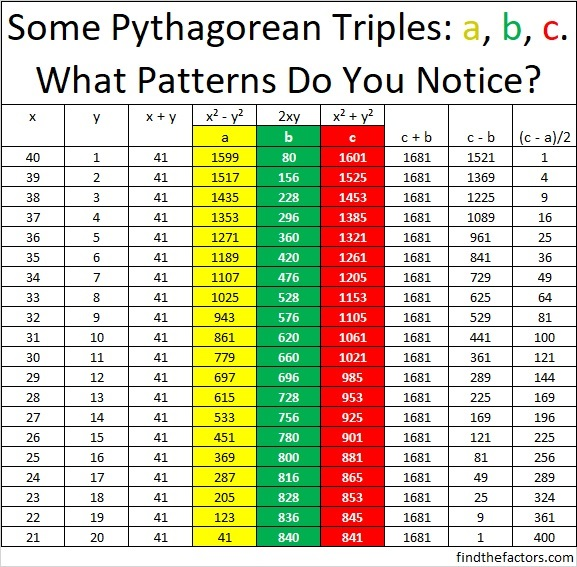Mathematics is truly a thing of beauty!

### Factors of 1681:

• 1681 is a composite number and is a perfect square.
• Prime factorization: 1681 = 41 × 41 which can be written 1681 = 41².
• 1681 has at least one exponent greater than 1 in its prime factorization so √1681 can be simplified. Taking the factor pair from the factor pair table below with the largest square number factor, we get √1681 = (√41)(√41) =
• The exponent in the prime factorization is 2. Adding one to that exponent, we get (2 + 1) = 3. Therefore 1681 has exactly 3 factors.
• The factors of 1681 are outlined with their factor pair partners in the graphic below.### More About the Number 1681:

1681 is the sum of two squares:
40² + 9² = 1681.

1681 is the hypotenuse of two Pythagorean triples:
369-1640-1681, which is 41 times (9-40-41).
720-1519-1681, calculated from 2(40)(9), 40² – 9², 40² + 9².

# 1089 Perfect Squares

The nine clues in today’s puzzle are all perfect squares. They are all you need to find all the factors that can turn this puzzle into a multiplication table . . . but with the rows and columns not in the typical order:Print the puzzles or type the solution in this excel file: 10-factors-1087-1094

1089 is also a perfect square.

The previous perfect square was 32² = (33 – 1)² = 33² + 1 – 2(33) = 1024
The next perfect square will be 34² = (33 + 1)² = 33² + 1 + 2(33) = 1156

Here’s a little more about the number 1089:

• 1089 is a composite number.
• Prime factorization: 1089 = 3 × 3 × 11 × 11, which can be written 1089 = 3²× 11²
• The exponents in the prime factorization are 2 and 2. Adding one to each and multiplying we get (2 + 1)(2 + 1) = 3 × 3 = 9. Therefore 1089 has exactly 9 factors.
• Factors of 1089: 1, 3, 9, 11, 33, 99, 121, 363, 1089
• Factor pairs: 1089 = 1 × 1089, 3 × 363, 9 × 121, 11 × 99, or 33 × 33
• 1089 is a perfect square. √1089 = 33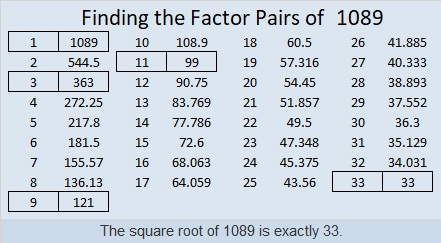1, 9, 121, and 1089 are all perfect square factors of 1089.

1089 can be 3 × 3 perfect squares arranged on an 11 × 11 perfect square grid:1089 can also be 11 × 11 perfect squares arranged on a 3 × 3 perfect square grid:Not only is 1089 the 33rd perfect square, but it is also the sum of the first 33 odd numbers. Note that the nth perfect square is also the sum of the first odd numbers: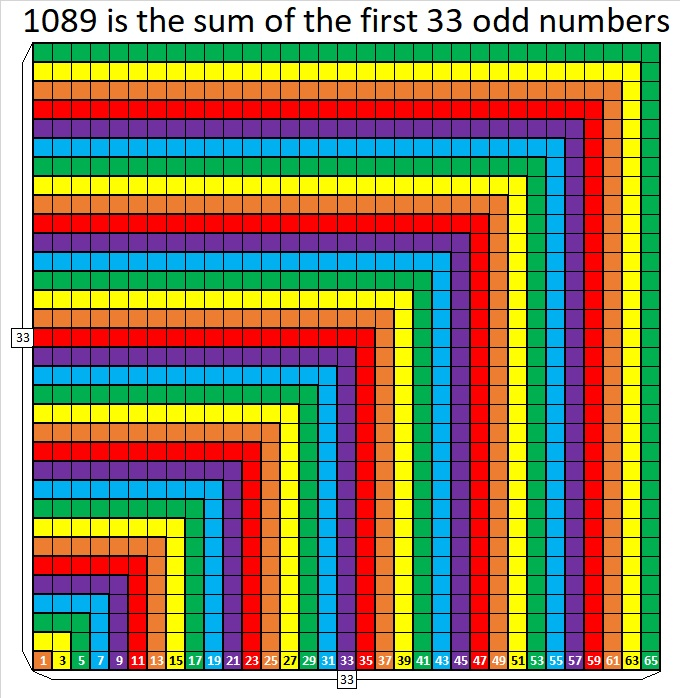I’m not attempting to make a picture of this nine-sided shape, but 1089 is the 18th nonagonal number because 18(7(18) – 5)/ 2 = 1089,
or written another way 7(18²)/2 – 5(18)/2 = 1089.

1089 is the sum of five consecutive prime numbers:
199 + 211 + 223 + 227 + 229 = 1089

OEIS.org informs us that 9 × 1089 = 9801

1089 looks rather square when it is written in several other bases:
It’s 900 in BASE 11 because 9(11²) = 1089,
441 in BASE 16 because  4(16²) + 4(16) + 1(1) = 1089,
169 in BASE 30 because 1(30²) + 6(30) + 9(1) = 1089,
144 in BASE 31 because 1(31²) + 4(31) + 4(1) = 1089,
121 in BASE 32 because 1(32²) + 2(32) + 1(1) = 1089,
100 in BASE 33 because 1(33²) = 1089

# 729 What I Did for Paula Krieg’s Star Project

Let me tell you a little about Paula Krieg’s Star Project:

The 12-Fold Rosette has its origin in Islamic Geometry. Paula Krieg is asking that people from a variety of backgrounds color in a section of the rosette and send it back to her so that she can assemble the different pieces into one lovely unified rosette. We are all stars, and some of the pieces look like stars, so she calls it her star project. You are invited to become a part of the project as well. Just click on this invitation to learn more. You can get a tile by either leaving a comment or e-mailing her a request, but do it soon. You will need to return your tile to her by 10 January 2016 to be included in the project.Rosette from Paula Krieg’s blog showing some possible coloring schemes for the tiles.

The piece that Paula sent me looked like this:I thought I would color it black, gray, white, and red because I think they look good together.

Since I don’t have any crayons in my house right now, and all my coloring pencils need to be sharpened, I decided to color it on my computer using the program Paint.

I brought up the e-mail Paula, opened the attachment, hit “Print Screen”, copied and pasted it into Paint, then cropped it.

I soon discovered that I could color in my entire tile using only black and gray and stay true to a goal I had given myself. The very tiny triangles insisted on staying white, but if I had increased the view size before I hit “Print Screen”, I would have been able to color them, too.I saved my coloring, but immediately felt like it really should have some red in it. I brought up my saved work, clicked on “save as”, named it “red tile”, and added some red. However, saving the file changed how it now received color. When I added some red, it looked like this, and I liked it and saved it again: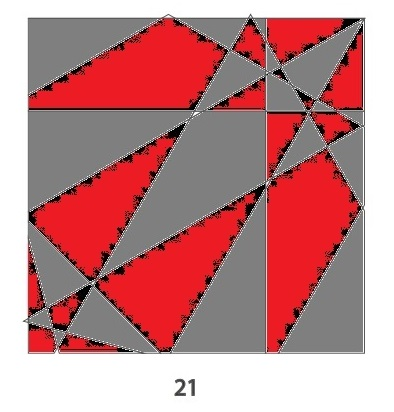Then I thought I might want a little more white, so I brought it back up and did “save as” again before adding some. This time it seem to allow even less additional color in each shape. (In other words, we see much more gray than black.)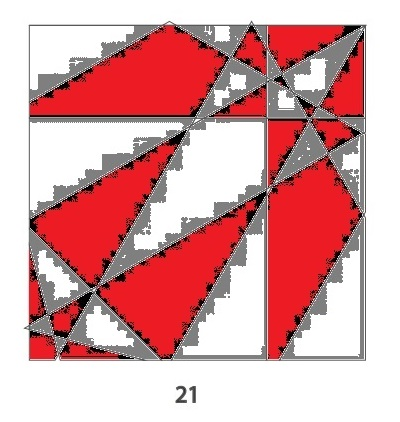I had so much fun doing this project. I have never considered myself to be much of an artist, but I felt a little artistic doing this project. I like all three tiles, but I think I like the final product the best.

You should join the project, too! You’re a star! Go ahead and shine! Whatever colors you use or whatever method you use to color your tile, your efforts will be appreciated. Also, because everyone is unique, every tile will look at least a little different. No tile will be ordinary.

Likewise, you might think that 729 is just an ordinary number, but much depends on how you look at it, too. Here are 729 equally sized squares.There’s a variety of ways to count the squares. Some ways remind us that 729 is a perfect square, other ways reminds us 729 is a perfect cube, and perhaps you can even find a way to see that 729 is a perfect 6th power, too.729 can be written as the sum of consecutive numbers several different ways:

• 364 + 365 = 729; that’s 2 consecutive numbers.
• 242 + 243 + 244 = 729; that’s 3 consecutive numbers.
• 119 + 120 + 121 + 122 + 123 + 124 = 729; that’s 6 consecutive numbers.
• 77 + 78 + 79 + 80 + 81 + 82 + 83 + 84 + 85 = 729; that’s 9 consecutive numbers.
• 32 + 33 + 34 + 35 + 36 + 37 + 38 + 39 + 40 + 41 + 42 + 43 + 44 + 45 + 46 + 47 + 48 + 49 = 729; that’s 18 consecutive numbers.
• 14 + 15 + 16 + 17 + 18 + 19 + 20 + 21 + 22 + 23 + 24 + 25 + 26 + 27 + 28 + 29 + 30 + 31 + 32 + 33 + 34 + 35 + 36 + 37 + 38 + 39 + 40 = 729; that’s 27 consecutive numbers.

729 is 1,000,000 in base 3, and 1000 in base 9, and 100 in base 27.

729 is also a palindrome in two bases:

• 1331 BASE 8; note that 1(512) + 3(64) + 3(8) + 1(1) = 729.
• 121 BASE 26; note that 1(26^2) + 2(26) + 1(1) = 729.

It is interesting that one less than 9 is 8, and one less than 27 is 26, and those are the two bases in which 729 is a palindrome!

Here is the factoring information for the number 729:

• 729 is a composite number. 729 = 27^2; 729 = 9^3; and 729 = 3^6
• Prime factorization: 729 = 3 x 3 x 3 x 3 x 3 x 3, which can be written 729 = (3^6)
• The exponent in the prime factorization is 6. Adding one we get (6 + 1) = 7. Therefore 729 has exactly 7 factors.
• Factors of 729: 1, 3, 9, 27, 81, 243, 729
• Factor pairs: 729 = 1 x 729, 3 x 243, 9 x 81, or 27 x 27
• 729 is a perfect square √729 = 27.# 676 and Level 3

676 is a palindrome in three consecutive different bases plus a couple of other bases:

• 10201 in base 5; note that 1(625) + 0(125) + 2(25) + 0(5) + 1(1) = 676.
• 676 in base 10; note that 6(100) + 7(10) + 6(1) = 676.
• 565 in base 11; note that 5(121) + 6(11) + 5(1) = 676.
• 484 in base 12; note that 4(144) + 8(12) + 4(1) = 676.
• 121 in base 25; note that 1(625) + 2(25) + 1(1) = 676.

Speaking of palindromes, OEIS.org states that 676 is the smallest perfect square palindrome whose square root is not also a palindrome. (Palindromic perfect squares less than 676 are 1, 4, 9, 121, and 484.)

Since 13 and 169 are two of its factors, 676 is also the hypotenuse of Pythagorean triples 476-480-676 and 260-624-676. What is the greatest common factor of each triple?Print the puzzles or type the solution on this excel file: 10 Factors 2015-11-09

—————————————————————————————————

• 676 is a composite number.
• Prime factorization: 676 = 2 x 2 x 13 x 13, which can be written 676 = (2^2) x (13^2)
• The exponents in the prime factorization are 2 and 2. Adding one to each and multiplying we get (2 + 1)(2 + 1) = 3 x 3 = 9. Therefore 676 has exactly 9 factors.
• Factors of 676: 1, 2, 4, 13, 26, 52, 169, 338, 676
• Factor pairs: 676 = 1 x 676, 2 x 338, 4 x 169, 13 x 52, or 26 x 26
• 676 is a perfect square. √676 = 26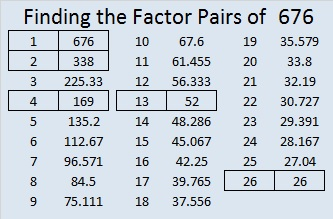—————————————————————————————————

A Logical Approach to solve a FIND THE FACTORS puzzle: Find the column or row with two clues and find their common factor. (None of the factors are greater than 10.)  Write the corresponding factors in the factor column (1st column) and factor row (top row).  Because this is a level three puzzle, you have now written a factor at the top of the factor column. Continue to work from the top of the factor column to the bottom, finding factors and filling in the factor column and the factor row one cell at a time as you go.# 576 and Level 5

The number formed from the last 2 digits of 576 is divisible by 4. That means 576 is also divisible by 4.

576 is made from 3 consecutive numbers so it is divisible by 3. Since the middle number, 6, is also divisible by 3, we know that 576 is also divisible by 9.

Either of the previous statements is enough to indicate that √576 can be reduced. In fact, boy, can it ever be reduced!

576 is the smallest number to have exactly 21 factors. (Only perfect squares have an odd number of factors.)

576 is also the sum of consecutive primes in two different ways:

• 283 + 293 = 576
• 137 + 139 + 149 + 151 = 576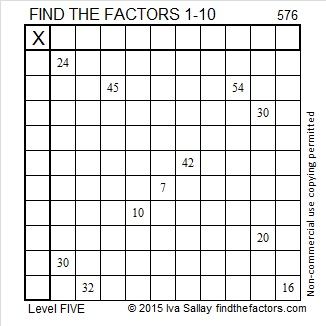Print the puzzles or type the solution on this excel file: 10 Factors 2015-08-03

—————————————————————————————————

• 576 is a composite number.
• Prime factorization: 576 = 2 x 2 x 2 x 2 x 2 x 2 x 3 x 3, which can be written 576 = (2^6) x (3^2)
• The exponents in the prime factorization are 6 and 2. Adding one to each and multiplying we get (6 + 1)(2 + 1) = 7 x 3 = 21. Therefore 576 has exactly 21 factors.
• Factors of 576: 1, 2, 3, 4, 6, 8, 9, 12, 16, 18, 24, 32, 36, 48, 64, 72, 96, 144, 192, 288, 576
• Factor pairs: 576 = 1 x 576, 2 x 288, 3 x 192, 4 x 144, 6 x 96, 8 x 72, 9 x 64, 12 x 48, 16 x 36, 18 x 32 or 24 x 24
• 576 is a perfect square. √576 = 24—————————————————————————————————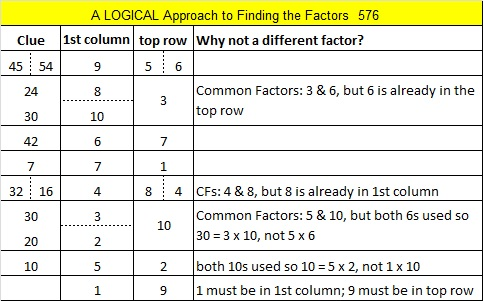# Is 529 an Interesting Number?

• 529 is a composite number.
• Prime factorization: 529 = 23^2
• The exponent in the prime factorization is 2. Adding one we get (2 + 1) = 3. Therefore 529 has exactly 3 factors.
• Factors of 529: 1, 23, 529
• Factor pairs: 529 = 1 x 529 or 23 x 23
• 529 is a perfect square. √529 = 23In the United States you can save money for college in a tax-free 529 College Savings Plan. If you use a 529 Plan, when you are 23, you could be making a good, square income. That is how I remember that 529 = 23².

529 dots can be made into a square, but is 529 an interesting number?

23 is the 9th prime number, and 529 is the 9th number with exactly 3 factors. Is that interesting?

What about the fact that 529 is palindrome 121 in BASE 22 because 1(22²) + 2(22) + 1(1) = 529?

Or that 529 can be written as the sum of three squares three ways and each of those ways contain exactly TWO elements of the set {18², , } plus one other square number?

• 22² + + = 529
• 18² + 14² + = 529
• 18² + 13² + = 529

Wikipedia informs us that 529 is the 12th centered octagonal number because  4(12²) – 4(12) + 1 = 529.

Do any of those reasons make 529 an interesting number? Is ANY number interesting?

Recently when I wrote that 526 is a centered pentagonal number, Steve Morris of Blog, Blogger, Bloggest commented:

It’s intriguing how so many numbers have interesting or special properties. I used to think that there was something magical about this, that these patterns were somehow telling us something deep about the universe. Like, why is 3.14159… the value it is?

Nowadays I think that these special properties are things we invented. For instance, a centred pentagonal number is interesting if you think it is, and isn’t if you don’t think so. Some numbers have particularly important or curious properties (prime numbers for instance), but they are only interesting because we think they are!

What do you think?

I think that much of mathematics was discovered by astronomers, physicists, and even philosophers, and the patterns in mathematics do tell us something deep about the universe, but all of those mathematical properties existed before they were discovered. They are eternal principles that we each understand to the best of our abilities.

Are numbers with certain properties interesting only because some people think they are?

If the world never knew that pi is approximately equal to 3.14159…, we would still be as advanced as we are now. Pi seems like a very important number, but what if the world had never heard of pi? What if for thousands of years the world had instead used tau (τ ) which is approximately equal to 6.28318? Every important, magical discovery related to pi would still be known. Since τ = 2π, some people think we should all celebrate on June 28 by eating twice as much pie. Those people are really saying that tau is an interesting number only because pi is interesting. If we had never heard of pi, we wouldn’t eat pie to celebrate either day. As it is, most people have never heard of τ and would consider it to be a rather boring number.

Are any numbers inherently interesting?

Ancient mathematicians were fascinated that a series of dots could be made into different shapes. Sometimes a certain number of dots could be made into a triangle, or a square, or even a pentagon. A string of dots that couldn’t be made into a 2-dimensional rectangle represented a prime number. The fact that the ancients were interested in the shape of numbers makes them even more interesting to me.

It doesn’t matter what language you use or even what number base you use, 529 ♦’s can be arranged into a perfectly formed square just as ♦ ♦ ♦ ♦ can be. Most people can understand square numbers or even cubed numbers. However, for some people triangular numbers and pentagonal numbers are just mind-boggling, and they won’t consider such numbers to be interesting at all.

I don’t know if the ancients were aware of CENTERED pentagonal numbers, but I am fascinated by the fact that this is a shape that CAN consist of a prime number of dots (31, 181, 331, for example.)

My husband often says that nothing is boring. Two different people can listen to the same talk. One of the listeners might be moved to tears or inspired to action while the other person is bored out of his mind. It isn’t the subject matter that decides how people will react, it’s the people themselves.

Mathematician G. H. Hardy is an inspiration to me. He said, “Nothing I have ever done is of the slightest practical use.” He studied mathematical topics that interested him without regard to their usefulness. How could he potentially waste Ramanujan’s great mind on something as useless as partition theory. How did he convince Cambridge University to pay him while he obsessively explored this unimportant topic especially when he boasted that it didn’t have the slightest practical use? I don’t know how he did that, but it turns out that partition theory was not simply recreational mathematics; it does have a practical purpose! From G. H. Hardy I have learned that it is okay to explore topics that interest me even if they NEVER have a practical purpose. I haven’t figured out how to get someone to pay me to explore any unimportant topics, but I can still explore them to my heart’s content:

I often notice if the square root of a number can be reduced or not. I did not invent the idea of reducing square roots, but I’ve decided it is an interesting topic. I don’t know if anybody else looks for consecutive reducible square roots or calculates what percentage of numbers have reducible square roots, but I find it interesting, and I explore it. If you google reducible square roots, chances are that much of what you see will have been created by me.

Some mathematicians spend time trying to prove the Riemann hypothesis, but that topic doesn’t interest me as much as other topics because that is what I’ve decided.

What do you think? What makes a number interesting or not? What makes a mathematical topic interesting or not?

# 441 Consecutive Numbers

The first 6 triangular numbers are

• 1 = 1
• 1 + 2 = 3
• 1 + 2 + 3 = 6
• 1 + 2 + 3 + 4 = 10
• 1 + 2 + 3 + 4 + 5 = 15
• 1 + 2 + 3 + 4 + 5 + 6 = 21

For millennia mathematicians have thought triangular numbers were quite interesting. 21 is a triangular number and 21 x 21 = 441 which makes 441 interesting, too. But wait, there’s something else that is very interesting about triangular numbers: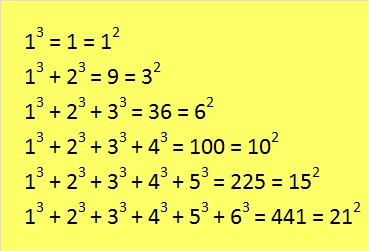It is amazing that when we begin with 1 cube, the sum of n consecutive cubes equals the nth triangular number squared every time!

What  follows is less amazing, but very practical. We can add consecutive numbers several ways to get 441. To find those ways we need to know the factors of 441.

• 441 is a composite number.
• Prime factorization: 441 = 3 x 3 x 7 x 7, which can be written 441 = (3^2) x (7^2)
• The exponents in the prime factorization are 2 and 2. Adding one to each and multiplying we get (2 + 1)(2 + 1) = 3 x 3 = 9. Therefore 441 has exactly 9 factors.
• Factors of 441: 1, 3, 7, 9, 21, 49, 63, 147, 441
• Factor pairs: 441 = 1 x 441, 3 x 147, 7 x 63, 9 x 49, or 21 x 21
• 441 is a perfect square. √441 = 21Because all of the factors for 441 are odd numbers, it is so easy to find consecutive numbers whose sum equal 441. Check out all of these:If we allowed negative numbers in the list of consecutive numbers we could also see that 441 equals the sum of 147 consecutive numbers that are centered around the number 3, and 49 consecutive numbers that are centered around the number 7, and 63 consecutive numbers that are centered around the number 9. All of those sums would be quite long.

Here are some more reasonable-length sums using only consecutive ODD numbers.That last sum reminds us that we always get n squared when we begin with one and add n consecutive odd numbers together.

# 361 Do You See a Pattern?

When 2^361 is divided by 361, the remainder is 116, not 2. That means that 361 is definitely a composite number. Its factors are listed at the end of this post.

361 isn’t used as often, but it is just as special as some of the numbers in the table below:The pattern can also be seen along the diagonals in this ordinary multiplication table:This pattern could be very helpful to students who are learning to multiply. I have seen plenty of students who knew 7 x 7 = 49, but couldn’t remember what 6 x 8 is.

Years after I learned the multiplication facts, I learned how to multiply binomials in an algebra class. I learned about the difference of two squares. In the example below one of the squares is n² and the other square is 1² which is equal to 1. I learned that the equation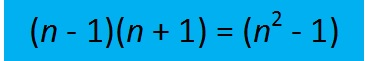is true for ALL numbers, but nobody pointed out any practical examples to make it more meaningful. The table at the top of the page contains twelve practical examples. Let’s see how you do applying it to products of a few larger numbers.

Sometimes we find easy ways to remember certain products like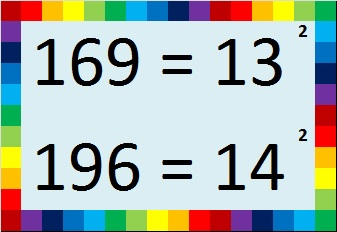We can use those products to help us remember other products easily by applying the difference of two squares. Try these: (Yes, you can easily do them without a calculator!)

• 13 x 13 = 169. How much is 12 x 14?
• 14 x 14 = 196. How much is 13 x 15?
• 20 x 20 = 400. How much is 19 x 21?
• If you know that the first ten powers of 2 are 2, 4, 8, 16, 32, 64, 128, 256, 512, 1024, then it’s easy to remember that 16 x 16 = 256. How much is 15 x 17?
• What if we go in the opposite direction…It isn’t too hard to multiply 18 x 20 in your head to get 360. How much is 19 x 19?
• 22 x 20 = 440 was also easy to find. How much is 21 x 21?
• 30 x 30 = 900. How much is 29 x 31?
• 100 x 100 = 10,000. How much is 99 x 101?Did you figure out what 361 has to do with this pattern? It is a perfect square just like 1, 4, 9, 16, and 25. Here is its factoring information:

• 361 is a composite number.
• Prime factorization: 361 = 19^2
• The exponent in the prime factorization is 2. Adding one we get (2 + 1) = 3. Therefore 361 has exactly 3 factors.
• Factors of 361: 1, 19, 361
• Factor pairs: 361 = 1 x 361 or 19 x 19
• 361 is a perfect square. √361 = 19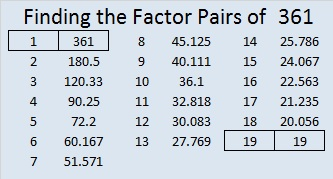# 289 and Level 6

• 289 is a composite number.
• Prime factorization: 289 = 17 x 17, which can be written 289 = (17^2)
• The exponent in the prime factorization is 2. Adding one we get (2 + 1) =  3. Therefore 289 has exactly 3 factors.
• Factors of 289: 1, 17, 289
• Factor pairs: 289 = 1 x 289 or 17 x 17
• 289 is a perfect square. √289 = 17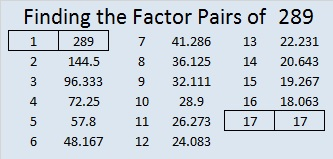1 + 3 + 5 + 7 + 9 + 11 + … + 29 + 31 + 33 = 289

Teachfurthermaths is now including Find the Factors in its list of useful links!Print the puzzles or type the factors on this excel file: 12 Factors 2014-11-03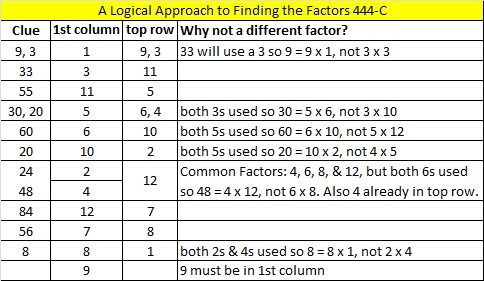# 225 and Level 6

• 225 is a composite number and a perfect square.
• Prime factorization: 225 = 3 x 3 x 5 x 5, which can be written 225 = (3^2) x (5^2)
• The exponents in the prime factorization are 2 and 2. Adding one to each and multiplying we get (2 + 1)(2 + 1) = 3 x 3 = 9. Therefore 225 has 9 factors.
• Factors of 225: 1, 3, 5, 9, 15, 25, 45, 75, 225
• Factor pairs: 225 = 1 x 225, 3 x 75, 5 x 45, 9 x 25, or 15 x 15
• √225 = 15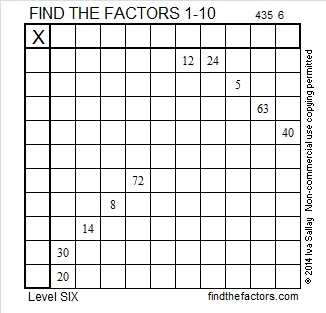Excel file of puzzles and previous week’s factor solutions: 10 Factors 2014-09-01